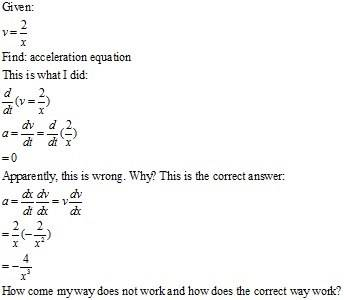# Derivative Chain Rule Question

Hi,

Say x=position, v=velocity, a=acceleration, t=time.Thanks!

EDIT: I just realized that 2/x is not a constant and thus I shouldn't have treated it as a constant (taking the derivative of it as 0). However, I don't understand how to take the derivative with respect to t of it.

Mark44
Mentor
Hi,

Say x=position, v=velocity, a=acceleration, t=time.Thanks!

EDIT: I just realized that 2/x is not a constant and thus I shouldn't have treated it as a constant (taking the derivative of it as 0). However, I don't understand how to take the derivative with respect to t of it.

Use the chain rule.
d/dt(2/x) = d/dx(2/x) * dx/dt

If you work through this, you'll get what you show as the correct answer.

By definition the time derivative of position is velocity.

Ugh, I forgot how to use the chain rule *slaps forehead*. I get it now. It was hard to visualize 2/x as a "function within a function".

Thanks guys!